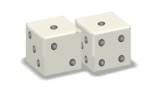Chapter 14.2, Problem 57E### Algebra and Trigonometry (MindTap ...

4th Edition
James Stewart + 2 others
ISBN: 9781305071742

#### Solutions

Chapter
Section### Algebra and Trigonometry (MindTap ...

4th Edition
James Stewart + 2 others
ISBN: 9781305071742
Textbook Problem

# APPLICATIONSSnake eyes What is the probability of rolling snakes eyes (“double ones”) three times in a row?To determine

To find:

The probability of rolling snakes eyes (“double ones”) three times in a row.

Explanation

Approach:

P(E)=n(E)n(S)(1)

Here, P(E) is the probability of the event E.

n(E) is the number of elements in E.

n(S) is the number of elements in the sample space S.

Fundamental principle of counting:

Suppose an event E occur in p different ways and an event F occur in q different ways then, the total number of ways of occurrence of these two events in the given order is p×q.

Calculation:

Let E be the event “rolling double ones”

### Still sussing out bartleby?

Check out a sample textbook solution.

See a sample solution

#### The Solution to Your Study Problems

Bartleby provides explanations to thousands of textbook problems written by our experts, many with advanced degrees!

Get Started